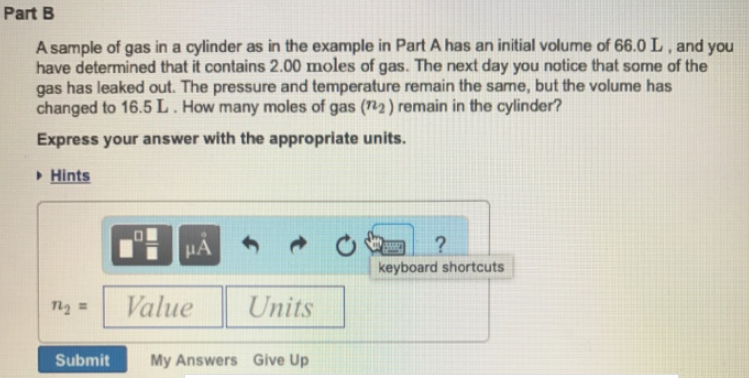# Problem: A sample of gas in a cylinder as in the example in Part A has an initial volume of 66.0 L, and you have determined that it contains 2.00 moles of gas. The next day you notice that some of the gas has leaked out. The pressure and temperature remain the same, but the volume has changed to 16.5 L. How many moles of gas (n2) remain in the cylinder? Express your answer with the appropriate units.

###### FREE Expert Solution
86% (132 ratings)###### Problem Details

A sample of gas in a cylinder as in the example in Part A has an initial volume of 66.0 L, and you have determined that it contains 2.00 moles of gas. The next day you notice that some of the gas has leaked out. The pressure and temperature remain the same, but the volume has changed to 16.5 L. How many moles of gas (n2) remain in the cylinder?